TitleCollege Algebra
Answer/Discussion to Practice Problems
Tutorial 17: Quadratic Equations

WTAMU > Virtual Math Lab > College Algebra > Tutorial 17: Quadratic EquationsAnswer/Discussion to 1a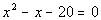Step 1: Simplify each side if needed.

 Step 2: Write in standard form,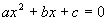, if needed.

 This quadratic equation is already in standard form.

 Step 3: Factor.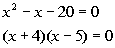*Quad. eq. in standard form *Factor the trinomial

 Step 4:  Use the Zero-Product Principle AND Step 5: Solve for the linear equation(s) set up in step 4.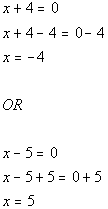*Use Zero-Product Principle *Solve the first linear equation           *Solve the second linear equation

 There are two solutions to this quadratic equation: x = -4 and x = 5.Answer/Discussion to 1b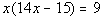Step 1: Simplify each side if needed.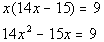*Use Dist. Prop. to clear the (  )

 Step 2: Write in standard form,, if needed.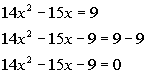*Inverse of add. 9 is sub. 9 *Quad. eq. in standard form

 Step 3: Factor.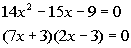*Quad. eq. in standard form *Factor the trinomial

 Step 4:  Use the Zero-Product Principle AND Step 5: Solve for the linear equation(s) set up in step 4.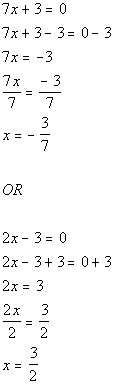*Use Zero-Product Principle *Solve the first linear equation                   *Solve the second linear equation

 There are two solutions to this quadratic equation: x = -3/7 and x = 3/2.Answer/Discussion to 2a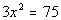Step 1: Write the quadratic equation in the form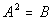if needed AND Step 2: Apply the square root method.

 Note how this quadratic equation is not in the formto begin with.  The 3 is NOT part of the expression being squared on the left side of the equation.  We can easily write it in the formby dividing both sides by 3.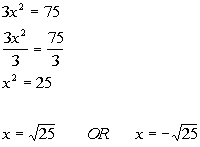*Not in the form*Inv. of mult. by 3 is div. by 3 *Written in the form*Apply the sq. root method *There are 2 solutions

 Step 3: Solve for the linear equation(s) set up in step 2.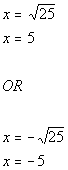*Sq. root of 25 = 5           *Neg. sq. root of 25 = -5

 There are two solutions to this quadratic equation: x = 5 and x = -5.Answer/Discussion to 2b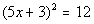Step 1: Write the quadratic equation in the formif needed AND Step 2: Apply the square root method.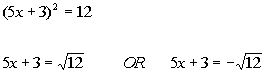*Written in the form*Apply the sq. root method *There are 2 solutions

 Step 3: Solve for the linear equation(s) set up in step 2.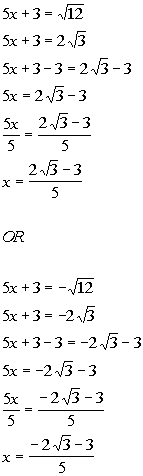*Sq. root of 12 = 2 sq. root of 3 *Solve for x                       *Neg. sq. root of 12 = -2 sq. root of 3 *Solve for x

 There are two solutions to this quadratic equation: x =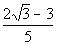and x =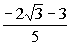.Answer/Discussion to 3a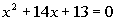Step 1: Make sure that the coefficient on the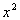term is equal to 1.

 The coefficient of theterm is already 1.

 Step 2:  Isolate theand x terms.

 Note how theand x terms are not isolated to begin with.  We can easily fix that by moving the constant to the other side of the equation.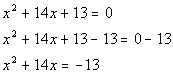*Inverse of add. 13 is sub. 13 *and x terms are now isolated

 Step 3:  Complete the square.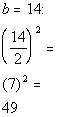*b is the coefficient of the x term *Complete the square by taking 1/2 of b and squaring it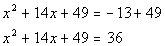*Add constant found above to BOTH sides of the eq. *This creates a PST on the left side of eq.

 Step 4:  Factor the perfect square trinomial (created in step 3) as a binomial squared.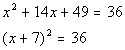*Factor the PST

 Step 5:  Solve the equation in step 4 by using the square root method.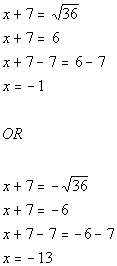*Written in the form*Apply the sq. root method *There are 2 solutions

 There are two solutions to this quadratic equation: x = -1  and x = -13.Answer/Discussion to 3b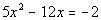Step 1:  Make sure that the coefficient on theterm is equal to 1.

 Note how the coefficient on theterm is not 1 to begin with.  We can easily fix that by dividing both sides by that coefficient, which in this case is 5 .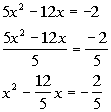*Divide both sides by 5   *Coefficient ofterm is now 1

 Step 2:  Isolate theand x terms.

 Theand x terms are already isolated.

 Step 3:  Complete the square.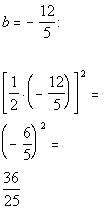*b is the coefficient of the x term     *Complete the square by taking 1/2 of b and squaring it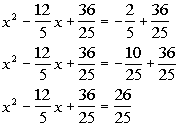*Add constant found above to BOTH sides of the eq.     *This creates a PST on the left side of eq.

 Step 4:  Factor the perfect square trinomial (created in step 3) as a binomial squared.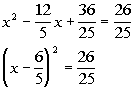*Factor the PST

 Step 5:  Solve the equation in step 4 by using the square root method.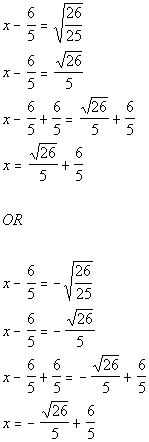*Written in the form*Apply the sq. root method *There are 2 solutions

 There are two solutions to this quadratic equation:  x =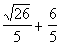and x =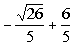.Answer/Discussion to 4a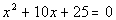Step 1: Simplify each side if needed.

 Step 2: Write in standard form,, if needed.

 This quadratic equation is already in standard form.

 Step 3: Identify a, b, and c.

 a, the number in front of x squared, is 1. b, the number in front of x, is 10.  c, the constant, is 25.   Make sure that you keep the sign that is in front of each of these numbers.  Next we will plug it into the quadratic formula.   Note that we are only plugging in numbers, we don't also plug in the variable.

 Step 4: Plug the values found in step 3 into the quadratic formula AND Step 5: Simplify if possible.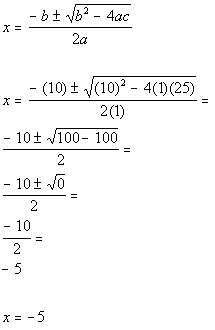*Quadratic formula     *Plug in values found above for a, b, and c *SimplifyAnswer/Discussion to 4b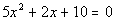Step 1: Simplify each side if needed.

 Step 2: Write in standard formif needed.

 This quadratic equation is already in standard form.

 Step 3: Identify a, b, and c.

 a, the number in front of x squared, is 5. b, the number in front of x, is 2.  c, the constant, is 10.   Make sure that you keep the sign that is in front of each of these numbers.  Next we will plug it into the quadratic formula.   Note that we are only plugging in numbers, we don't also plug in the variable.

 Step 4: Plug the values found in step 3 into the quadratic formula AND Step 5: Simplify if possible.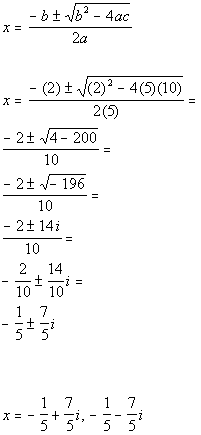*Quadratic formula     *Plug in values found above for a, b, and c *SimplifyAnswer/Discussion to 4c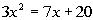Step 1: Simplify each side if needed.

 Step 2: Write in standard formif needed.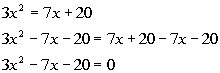*Inverse of add. 7x and 20 is sub. 7x and 20 *Quad. eq. in standard form

 Step 3: Identify a, b, and c.

 a, the number in front of x squared, is 3. b, the number in front of x, is -7.  c, the constant, is -20.   Make sure that you keep the sign that is in front of each of these numbers.  Next we will plug it into the quadratic formula.   Note that we are only plugging in numbers, we don't also plug in the variable.

 Step 4: Plug the values found in step 3 into the quadratic formula AND Step 5: Simplify if possible.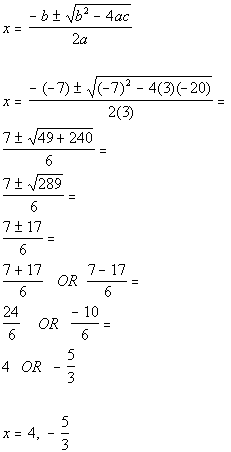*Quadratic formula       *Plug in values found above for a, b, and c *SimplifyAnswer/Discussion to 5a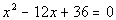Step 1: Simplify each side if needed.

 Step 2: Write in standard form,, if needed.

 This quadratic equation is already in standard form.

 Step 3: Identify a, b, and c.

 a, the number in front of x squared, is 1. b, the number in front of x, is -12. c, the constant, is 36.   Make sure that you keep the sign that is in front of each of these numbers.

 Step 4: Plug the values found in step 3 into the discriminant,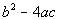, AND Step 5: Simplify if possible.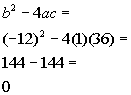*Discriminant formula *Plug in values found above for a, b, and c *Discriminant

 Since the discriminant is zero, that means there is only one real number solution.Answer/Discussion to 5b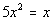Step 1: Simplify each side if needed.

 Step 2: Write in standard form,, if needed.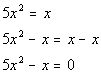*Inverse of add. x is sub. x *Quad. eq. in standard form

 Step 3: Identify a, b, and c.

 a, the number in front of x squared, is 5. b, the number in front of x, is -1. c, the constant, is 0. Note that since the constant is missing it is understood to be 0.   Make sure that you keep the sign that is in front of each of these numbers.

 Step 4: Plug the values found in step 3 into the discriminant,, AND Step 5: Simplify if possible.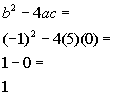*Discriminant formula *Plug in values found above for a, b, and c *Discriminant

 Since the discriminant is a positive number, that means there are two distinct real number solutions.Answer/Discussion to 5c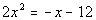Step 1: Simplify each side if needed.

 Step 2: Write in standard form,, if needed.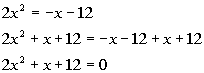*Inverse of sub. x and 12 is add. x and 12 *Quad. eq. in standard form

 Step 3: Identify a, b, and c.

 a, the number in front of x squared, is 2. b, the number in front of x, is 1. c, the constant, is 12.   Make sure that you keep the sign that is in front of each of these numbers.

 Step 4: Plug the values found in step 3 into the discriminant,, AND Step 5: Simplify if possible.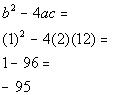*Discriminant formula *Plug in values found above for a, b, and c *Discriminant

 Since the discriminant is a negative number, that means there are two distinct complex imaginary solutions.

Last revised on Dec. 16, 2009 by Kim Seward.
All contents copyright (C) 2002 - 2010, WTAMU and Kim Seward. All rights reserved.